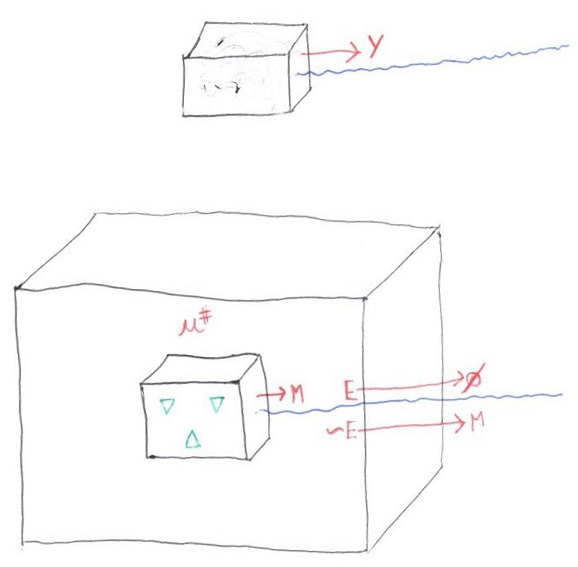# 7

Personal Blog

A putative new idea for AI control; index here.

This post summaries the methods for getting honest answers from certain AIs, presented in the previous two posts.

You can basically safely ask an AI for expected utility estimates (including conditional ones), probability estimates, and get the AI to identify events of highest and lowest probability and utility, and, arguably, highest and lowest conditional utility.

The setup is a boxed AI, which emits a message M, and a stochastic stochastic process E that would erase M with low probability, before anyone could see it. There is also a stochastic process that emits a message Y. Let v_E be the indicator function for E and v_y the indicator function for Y=y.Then, given a utility u bounded between 0 and 1, and a specific y, we can give the AI a utility u# that will cause it to give us the message M_1={q,q^y,p^y,q^{|y}} (which we will see with high probability P(¬E), an event the AI will not optimise for).

Define f(q,u)=-q^2 +2qu. The utility is then

• u#(1) = v_E(f(q,u) + f(q^y,uv_y) + f(p^y,v_y) + f(q^{|y},u)v_y).

Maximising this u# ensures that, in the AI's best estimate,

1. q = E(u),
2. q^y = E(u ∧ (Y=y)),
3. p^{y} = P(Y=y),
4. q^{|y} = q^{y}/p^{y}=E(u|Y=y).

But we need not consider any specific y. The AI could send out the message M_2={y^+,y^-,y^{p+},y^{p-},q^+,q^-,p^+,p^-}, while maximising the utility

• u#(2) = v_E (f(q^+,uv_{y^+}) + f(q^-,(1-u)v_{y^-}) + f(p^+,v_{y^{p+}}) + f(p^-,1-v_{y^{p-}})).

Then M will be such that, in the AI's best estimate,

1. (y^+,q^+) = (argmax_y, max_y) E(u ∧ (Y=y)),
2. (y^-,q^-) = (argmin_y, min_y) E(u ∧ (Y=y)),
3. (y^{p+},p^+) = (argmax_y, max_y) P(Y=y),
4. (y^{p-},p^-) = (argmin_y, min_y) P(Y=y),

Finally, define M_{3,r} = {y^{|+},y^{|-},q^{|+},q^{|-}}. And set f_r(q,u)= -(r-1)q^r+ rq^{r-1}u, and define the utility function:

• u#(3,r) = v_E v_{y^{|+}}f_r(q{|+},u) + v_E v_{y^{|-}}f_r(q^{|-},1-u).

Then, for large enough r, the AI will output M so that, according to its best estimate,

1. (y^{|+},q^{|+}) = (argmax_y, max_y) E(u|(Y=Y^+)),
2. (y^{|-},q^{|-}) = (argmin_y, min_y) E(u|(Y=Y^+)).

Unfortunately the value of r cannot be known ahead of time. It might be possible to define a "limit" utility u#(3, ∞).

Note that all these messages and utilities are independent, so the AI can generate messages M_1 + M_2 + M_{3,r} + M_{3,r'} when maximising

• u#(1) + u#(2) + u#(3,r) + u#(3,r').

But there are issues with very low probabilities, as explained in the previous post.

Personal Blog